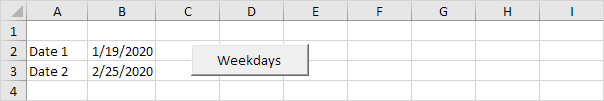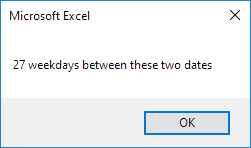# Weekdays

Below we will look at a program in Excel VBA that calculates the number of weekdays between two dates.

Weekdays are: Monday, Tuesday, Wednesday, Thursday and Friday.

Situation:Note: Dates are in US Format. Months first, Days Second. This type of format depends on your windows regional settings.

1. First, we declare five variables. date1 of type Date, date2 of type Date, dateToCheck of type Date, daysBetween of type Integer, weekdays of type Integer and i of type Integer.

Dim date1 As Date, date2 As Date, dateToCheck As Date
Dim daysBetween As Integer, weekdays As Integer, i As Integer

2. We initialize four variables. We use the DateDiff function to initialize the variable daysBetween. This function has three arguments. We fill in "d" for the first argument since we want the number of days between date1 and date2.

weekdays = 0
date1 = Range("B2")
date2 = Range("B3")
daysBetween = DateDiff("d", date1, date2)

3. We need to check for each date between date1 and date2 (including date1 and date2) whether the date is a weekday or not. If yes, we increment weekdays by 1. We will use a For Next loop.

For i = 0 To daysBetween

4. We use the DateAdd function to get each date we need to check. This function has three arguments. We fill in "d" for the first argument since we want to add days, i for the second argument, and date1 for the third argument since we want to add i days to date1. This way Excel VBA can check each date between date1 and date2 starting with date1. Add the following code line:

Example: for i = 3, Excel VBA checks date1 + 3 days.

5. Next, we use the Weekday function (build in function) to check whether dateToCheck is a weekday or not. The Weekday function returns 1 for a Sunday and 7 for a Saturday. Therefore, we only increment the variable weekdays if Weekday(dateToCheck) is not equal to 1 and not equal to 7 (<> means not equal to). The following code lines get the job done.

If (Weekday(dateToCheck) <> 1 And Weekday(dateToCheck) <> 7) Then
weekdays = weekdays + 1
End If

6. Don't forget to close the loop.

Next i

7. Finally, we display the number of weekdays using a MsgBox. We use the & operator to concatenate (join) two strings. Although weekdays is not a string it works here.

MsgBox weekdays & " weekdays between these two dates"

8. Place your macro in a command button and test it.

Result:Go to Next Chapter: Events